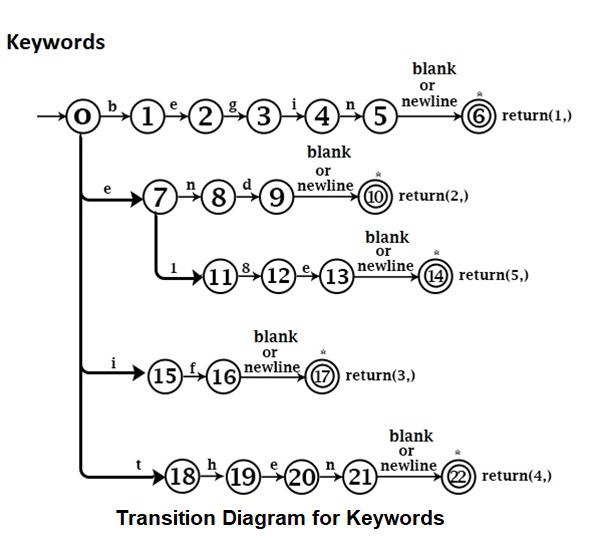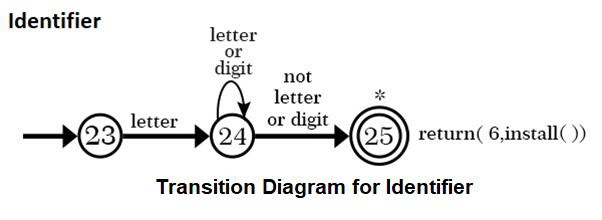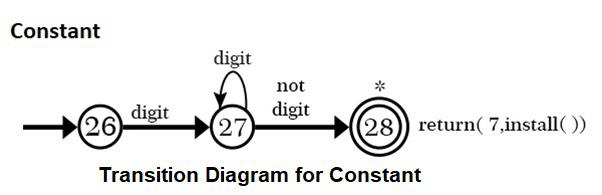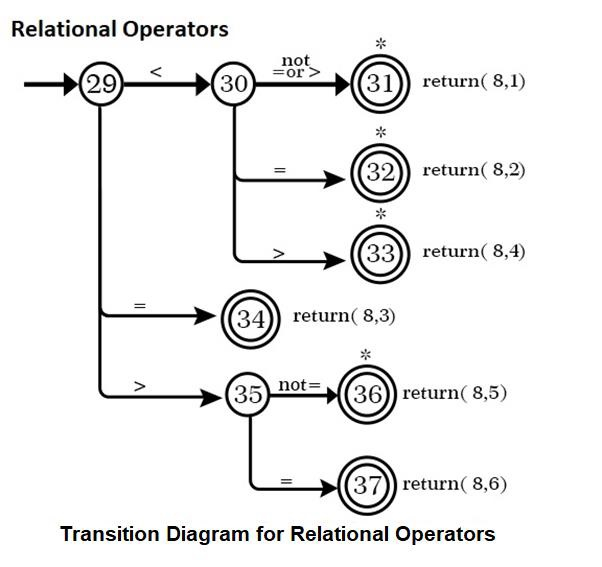# What is Design of Lexical Analysis in Compiler Design?

Lexical Analysis can be designed using Transition Diagrams.

Finite Automata (Transition Diagram) − A Directed Graph or flowchart used to recognize token.

The transition Diagram has two parts −

• States − It is represented by circles.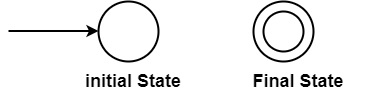• Edges − States are connected by Edges Arrows.

Example − Draw Transition Diagram for "if" keyword.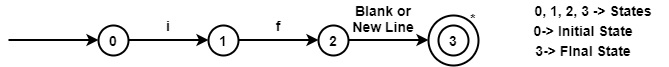To recognize Token ("if"), Lexical Analysis has to read also the next character after "f". Depending upon the next character, it will judge whether the "if" keyword or something else is.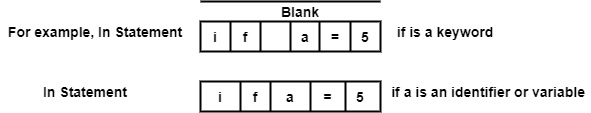So, Blank space after "if" determines that "If" is a keyword.

"*" on Final State 3 means Retract, i.e., control will again come to previous state 2. Therefore Blank space is not a part of the Token ("if").

Transition Diagram for an Identifier − An identifier starts with a letter followed by letters or Digits. Transition Diagram will be: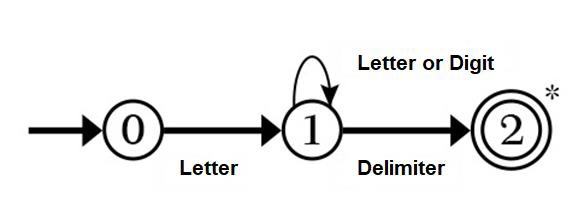For example, In statement int a2; Transition Diagram for identifier a2 will be: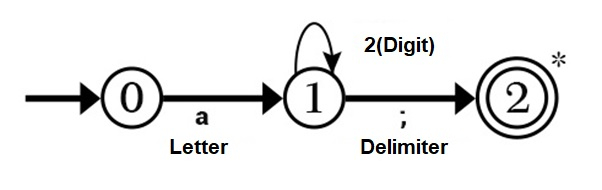As (;) is not part of Identifier ("a2"), so use "*" for Retract i.e., coming back to state 1 to recognize identifier ("a2").

The Transition Diagram for identifier can be converted to Program Code as −

Coding

State 0: C = Getchar()
If letter (C) then goto state 1 else fail

State1: C = Getchar()
If letter (C) or Digit (C) then goto state 1
else if Delimiter (C) goto state 2
else Fail

State2: Retract ()
return (6, Install ());

In-state 2, Retract () will take the pointer one state back, i.e., to state 1 & declares that whatever has been found till state 1 is a token.

The lexical Analysis will return the token to the Parser, not in the form of an English word but the form of a pair, i.e., (Integer code, value).

In the case of identifier, the integer code returned to the parser is 6 as shown in the table.

Install () − It will return a pointer to the symbol table, i.e., address of tokens.

The following table shows the integer code and value of various tokens returned by lexical analysis to the parser.

Integer Codes for different Tokens

TokenInteger CodeValue
Begin1-
End2-
If3-
Then4-
Else5-
Identifier6Pointer to Symbol Table
Constants7Pointer to Symbol Table
<81
<=82
=83
<>84
>85
>=86

These integer values are not fixed. Different Programmers can choose other integer codes and values while designing the Lexical Analysis.

Suppose, if the identifier is stored at location 236 in the symbol table, then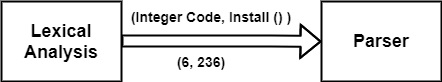Similarly, if constant is stored at location 238 then

Integer code = 7

Install () = 238 i.e., Pair will be (7, 238)

Transition Diagram (Finite Automata) for Tokens −